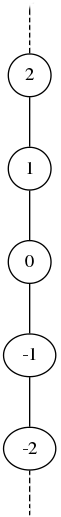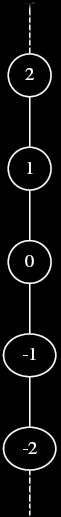# Poset: Examples

The standard $$\leq$$ relation on integers, the $$\subseteq$$ relation on sets, and the $$|$$ (divisibility) relation on natural numbers are all examples of poset orders.

# Integer Comparison

The set $$\mathbb Z$$ of integers, ordered by the standard “less than or equal to” operator $$\leq$$ forms a poset $$\langle \mathbb Z, \leq \rangle$$. This poset is somewhat boring however, because all pairs of elements are comparable; such posets are called chains or totally ordered sets. Here is its Hasse diagram.comment: dot source (doctored in GIMP)

digraph G { node = 0.1, height = 0.1 edge = “none” a = “-3″ b = “-2” c = “-1″ d = “0” e = “1″ f = “2” g = “3″ rankdir = BT; a → b b → c c → d d → e e → f f → g } <div>

# Power sets

For any set $$X$$, the power set of $$X$$ ordered by the set inclusion relation $$\subseteq$$ forms a poset $$\langle \mathcal{P}(X), \subseteq \rangle$$. $$\subseteq$$ is clearly reflexive, since any set is a subset of itself. For $$A,B \in \mathcal{P}(X)$$, $$A \subseteq B$$ and $$B \subseteq A$$ combine to give $$x \in A \Leftrightarrow x \in B$$ which means $$A = B$$. Thus, $$\subseteq$$ is antisymmetric. Finally, for $$A, B, C \in \mathcal{P}(X)$$, $$A \subseteq B$$ and $$B \subseteq C$$ give $$x \in A \Rightarrow x \in B$$ and $$x \in B \Rightarrow x \in C$$, and so the transitivity of $$\subseteq$$ follows from the transitivity of $$\Rightarrow$$.

Note that the strict subset relation $$\subset$$ is the strict ordering derived from the poset $$\langle \mathcal{P}(X), \subseteq \rangle$$.

# Divisibility on the natural numbers

Let $$\mathbb N$$ be the set of natural numbers including zero, and let $$|$$ be the divides relation, where $$a|b$$ whenever there exists an integer $$k$$ such that $$ak=b$$. Then $$\langle \mathbb{N}, | \rangle$$ is a poset.$$|$$ is reflexive because, letting k=1, any natural number divides itself. To see that $$|$$ is anti-symmetric, suppose $$a|b$$ and $$b|a$$. Then there exist integers $$k_1$$ and $$k_2$$ such that $$a = k_1b$$ and $$b = k_2a$$. By substitution, we have $$a = k_1k_2a$$. Thus, if either $$k$$ is $$0$$, then both $$a$$ and $$b$$ must be $$0$$. Otherwise, both $$k$$’s must equal $$1$$ so that $$a = k_1k_2a$$ holds. Either way, $$a = b$$, and so $$|$$ is anti-symmetric. To see that $$|$$ is transitive, suppose that $$a|b$$ and $$b|c$$. This implies the existence of integers $$k_1$$ and $$k_2$$ such that $$a = k_1b$$ and $$b = k_2c$$. Since by substitution $$a = k_1k_2c$$, we have $$a|c$$.

Parents: